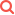### 1.Matrix Schedules#

Purpose

Use this procedure to set up matrices that can supply values for appraisals through Improvement Schedules.

The values assigned within a matrix can provide the unit cost of an improvement based on user criteria. Matrices can then supply modifiers and adjustments to the unit cost.

Note   This procedure is very similar to the procedure for setting up a land matrix.

Prerequisites

• The following user right is assigned to your user ID:
• PACS Administrator > Improvement Matrix Maintenance

Procedure

1. In PACS Appraisal, browse to PACS Application button, choose Tools > Schedule Maintenance > Matrix Maintenance > Improvement.
2. In the Matrix Maintenance Improvement window, click Add.Note    The matrix can only be created in the current appraisal year.

3. In the Description field, enter a brief description identifying the matrix you are creating.
4. Select the type of matrix being created from the Label drop-down list. Label options include the following:
• DEPR – used when the Matrix table is a depreciation table
• FEATURE_UC – Used when the Matrix table is designed to generate a value for a feature that displays in the feature listing, but not as part of the unit price for the detail
• MOD – Modifier – Used when the Matrix table is designed to modify the unit price of the improvement detail
• UC – Unit Cost – Used when the Matrix table is designed to determine the initial price for an improvement detail
• AF – Area Factor – Similar to MOD, simply put is an adjustment
• MOD_W_F-UNIT – Similar to AF and MOd, except the cell value is multiplied by the number of feature units before being applied and is therefore intended for matrices whose X axis is a feature (Mod_w_F_unit would NOT be used on a matrix whose axis are class and subclass, for example).

Note    A matrix designed to determine the unit cost of a parcel uses the label `UC`, for example. A matrix designed to modify the unit cost uses `MOD`, the modifier label.

5. Select a function from the Operator drop-down list. The operator determines how the values are applied. Operator options include the following:
• Addition – Used when the matrix is designed to add value to the unit price of the improvement detail
• GOTO – Used when the matrix is a depreciation table
• End – Used in depreciation table lookups
• MAX – When the current matrix evaluation results in a value greater than the current running value, then the current running value is replaced with said value; otherwise it is ignored
• Multiplicative – Used when the matrix table is designed to multiply the running total unit price by another value on the improvement detail
• Special – Used with depreciation table matrices as the first matrix in the list; all previous work is ignored. In PACS, running value equals the value from this matrix.
6. Select the properties to use in the matrix from the Axis 1 and Axis 2 drop-down lists. Then populate the axes by clicking the Data Type buttons and doing one of the following:• Click the Addbutton and enter values• Use the arrows to move codes from the Available column to the Selected column7. Set all of the cells within the grid to a starting or default value by entering the value in the Default Cell Value field.
8. To automatically calculate the percentage differential between each defined range, select Interpolate.

Tip   With Interpolate, the value change between ranges occurs gradually. As such, Interpolate should only be used when working with economies of scale, such as area.

9. Next, set the value of each cell by doing the following:
• Select the cell
• Enter the value
• Click Set Selected Cell Values or press the `Tab `key to automatically select the next cell in the grid

Tip    More than one cell value can be set at a time. Select multiple cells by pressing CTRL and clicking on cells.10. Select Print as needed.
11. Click OK or Apply.

WARNING! – Editing the values in a matrix can change the value of all properties to which the matrix is currently assigned.

Suggest Edit